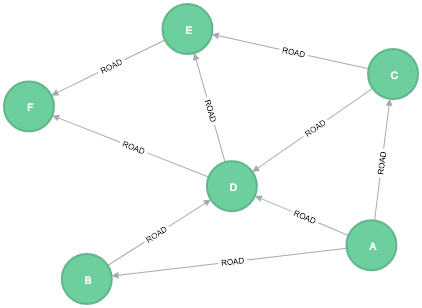# All Pairs Shortest Path

This feature is in the alpha tier. For more information on feature tiers, see API Tiers.

The All Pairs Shortest Path (APSP) calculates the shortest (weighted) path between all pairs of nodes. This algorithm has optimizations that make it quicker than calling the Single Source Shortest Path algorithm for every pair of nodes in the graph.

This feature is in the alpha tier. For more information on feature tiers, see API Tiers.

## 1. History and explanation

Some pairs of nodes might not be reachable between each other, so no shortest path exists between these pairs. In this scenario, the algorithm will return `Infinity` value as a result between these pairs of nodes.

Plain cypher does not support filtering `Infinity` values, so `gds.util.isFinite` function was added to help filter `Infinity` values from results.

## 2. Use-cases - when to use the All Pairs Shortest Path algorithm

• The All Pairs Shortest Path algorithm is used in urban service system problems, such as the location of urban facilities or the distribution or delivery of goods. One example of this is determining the traffic load expected on different segments of a transportation grid. For more information, see Urban Operations Research.

• All pairs shortest path is used as part of the REWIRE data center design algorithm that finds a network with maximum bandwidth and minimal latency. There are more details about this approach in "REWIRE: An Optimization-based Framework for Data Center Network Design"

## 3. Syntax

The following will run the algorithm and stream results:
``````CALL gds.alpha.allShortestPaths.stream(
graphName: string,
configuration: map
)
YIELD startNodeId, targetNodeId, distance``````
Table 1. Parameters
Name Type Default Optional Description

relationshipWeightProperty

String

null

yes

Name of the relationship property to use as weights. If unspecified, the algorithm runs unweighted.

concurrency

Integer

4

yes

The number of concurrent threads used for running the algorithm. Also provides the default value for 'readConcurrency' and 'writeConcurrency'. This is dependent on the Neo4j edition; for more information, see CPU.

Integer

value of 'concurrency'

yes

## 4. All Pairs Shortest Path algorithm sampleThe following will create a sample graph:
``````CREATE (a:Loc {name: 'A'}),
(b:Loc {name: 'B'}),
(c:Loc {name: 'C'}),
(d:Loc {name: 'D'}),
(e:Loc {name: 'E'}),
(f:Loc {name: 'F'}),
The following will project and store a graph using native projection:
``````CALL gds.graph.project(
'nativeGraph',
'Loc',
{
properties: 'cost'
}
}
)
YIELD graphName``````
The following will run the algorithm and stream results:
``````CALL gds.alpha.allShortestPaths.stream('nativeGraph', {
relationshipWeightProperty: 'cost'
})
YIELD sourceNodeId, targetNodeId, distance
WITH sourceNodeId, targetNodeId, distance
WHERE gds.util.isFinite(distance) = true

MATCH (source:Loc) WHERE id(source) = sourceNodeId
MATCH (target:Loc) WHERE id(target) = targetNodeId
WITH source, target, distance WHERE source <> target

RETURN source.name AS source, target.name AS target, distance
ORDER BY distance DESC, source ASC, target ASC
LIMIT 10``````
Table 2. Results
Source Target Cost

A

F

160

A

E

120

B

F

110

C

F

110

A

D

90

B

E

70

C

E

70

D

F

70

A

B

50

A

C

50

This query returned the top 10 pairs of nodes that are the furthest away from each other. F and E appear to be quite distant from the others.

For now, only single-source shortest path support loading the relationship as undirected, but we can use Cypher loading to help us solve this. Undirected graph can be represented as Bidirected graph, which is a directed graph in which the reverse of every relationship is also a relationship.

We do not have to save this reversed relationship, we can project it using Cypher loading. Note that relationship query does not specify direction of the relationship. This is applicable to all other algorithms that use Cypher loading.

The following will project and store an undirected graph using cypher projection:
``````CALL gds.graph.project.cypher(
'cypherGraph',
'MATCH (n:Loc) RETURN id(n) AS id',
'MATCH (n:Loc)-[r:ROAD]-(p:Loc) RETURN id(n) AS source, id(p) AS target, r.cost AS cost'
)
YIELD graphName``````
The following will run the algorithm, treating the graph as undirected:
``````CALL gds.alpha.allShortestPaths.stream('cypherGraph', {
relationshipWeightProperty: 'cost'
})
YIELD sourceNodeId, targetNodeId, distance
WITH sourceNodeId, targetNodeId, distance
WHERE gds.util.isFinite(distance) = true

MATCH (source:Loc) WHERE id(source) = sourceNodeId
MATCH (target:Loc) WHERE id(target) = targetNodeId
WITH source, target, distance WHERE source <> target

RETURN source.name AS source, target.name AS target, distance
ORDER BY distance DESC, source ASC, target ASC
LIMIT 10``````
Table 3. Results
Source Target Cost

A

F

160

F

A

160

A

E

120

E

A

120

B

F

110

C

F

110

F

B

110

F

C

110

A

D

90

D

A

90